# 3.5 Transformation of functions  (Page 11/21)

 Page 11 / 21

When examining the formula of a function that is the result of multiple transformations, how can you tell a horizontal stretch from a vertical stretch?

When examining the formula of a function that is the result of multiple transformations, how can you tell a horizontal compression from a vertical compression?

A horizontal compression results when a constant greater than 1 is multiplied by the input. A vertical compression results when a constant between 0 and 1 is multiplied by the output.

When examining the formula of a function that is the result of multiple transformations, how can you tell a reflection with respect to the x -axis from a reflection with respect to the y -axis?

How can you determine whether a function is odd or even from the formula of the function?

For a function $\text{\hspace{0.17em}}f,\text{\hspace{0.17em}}$ substitute $\text{\hspace{0.17em}}\left(-x\right)\text{\hspace{0.17em}}$ for $\text{\hspace{0.17em}}\left(x\right)\text{\hspace{0.17em}}$ in $\text{\hspace{0.17em}}f\left(x\right).\text{\hspace{0.17em}}$ Simplify. If the resulting function is the same as the original function, $\text{\hspace{0.17em}}f\left(-x\right)=f\left(x\right),\text{\hspace{0.17em}}$ then the function is even. If the resulting function is the opposite of the original function, $\text{\hspace{0.17em}}f\left(-x\right)=-f\left(x\right),\text{\hspace{0.17em}}$ then the original function is odd. If the function is not the same or the opposite, then the function is neither odd nor even.

## Algebraic

For the following exercises, write a formula for the function obtained when the graph is shifted as described.

$\text{\hspace{0.17em}}f\left(x\right)=\sqrt{x}\text{\hspace{0.17em}}$ is shifted up 1 unit and to the left 2 units.

$\text{\hspace{0.17em}}f\left(x\right)=|x|\text{\hspace{0.17em}}$ is shifted down 3 units and to the right 1 unit.

$g\left(x\right)=|x-1|-3$

$\text{\hspace{0.17em}}f\left(x\right)=\frac{1}{x}\text{\hspace{0.17em}}$ is shifted down 4 units and to the right 3 units.

$\text{\hspace{0.17em}}f\left(x\right)=\frac{1}{{x}^{2}}\text{\hspace{0.17em}}$ is shifted up 2 units and to the left 4 units.

$g\left(x\right)=\frac{1}{{\left(x+4\right)}^{2}}+2$

For the following exercises, describe how the graph of the function is a transformation of the graph of the original function $\text{\hspace{0.17em}}f.$

$y=f\left(x-49\right)$

$y=f\left(x+43\right)$

The graph of $\text{\hspace{0.17em}}f\left(x+43\right)\text{\hspace{0.17em}}$ is a horizontal shift to the left 43 units of the graph of $\text{\hspace{0.17em}}f.$

$y=f\left(x+3\right)$

$y=f\left(x-4\right)$

The graph of $\text{\hspace{0.17em}}f\left(x-4\right)\text{\hspace{0.17em}}$ is a horizontal shift to the right 4 units of the graph of $\text{\hspace{0.17em}}f.$

$y=f\left(x\right)+5$

$y=f\left(x\right)+8$

The graph of $\text{\hspace{0.17em}}f\left(x\right)+8\text{\hspace{0.17em}}$ is a vertical shift up 8 units of the graph of $\text{\hspace{0.17em}}f.$

$y=f\left(x\right)-2$

$y=f\left(x\right)-7$

The graph of $\text{\hspace{0.17em}}f\left(x\right)-7\text{\hspace{0.17em}}$ is a vertical shift down 7 units of the graph of $\text{\hspace{0.17em}}f.$

$y=f\left(x-2\right)+3$

$y=f\left(x+4\right)-1$

The graph of $f\left(x+4\right)-1$ is a horizontal shift to the left 4 units and a vertical shift down 1 unit of the graph of $f.$

For the following exercises, determine the interval(s) on which the function is increasing and decreasing.

$f\left(x\right)=4{\left(x+1\right)}^{2}-5$

$g\left(x\right)=5{\left(x+3\right)}^{2}-2$

decreasing on $\text{\hspace{0.17em}}\left(-\infty ,-3\right)\text{\hspace{0.17em}}$ and increasing on $\text{\hspace{0.17em}}\left(-3,\infty \right)$

$a\left(x\right)=\sqrt{-x+4}$

$k\left(x\right)=-3\sqrt{x}-1$

decreasing on $\left(0,\text{\hspace{0.17em}}\infty \right)$

## Graphical

For the following exercises, use the graph of $\text{\hspace{0.17em}}f\left(x\right)={2}^{x}\text{\hspace{0.17em}}$ shown in [link] to sketch a graph of each transformation of $\text{\hspace{0.17em}}f\left(x\right).$

$g\left(x\right)={2}^{x}+1$

$h\left(x\right)={2}^{x}-3$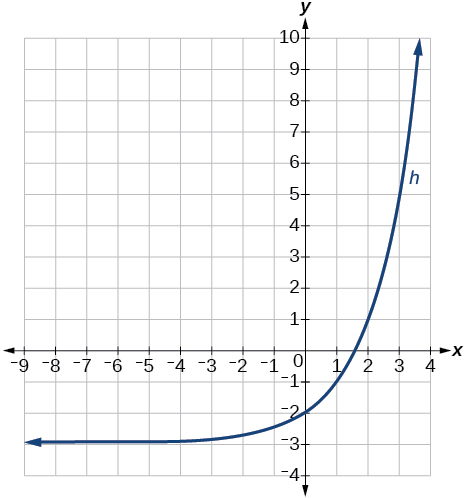$w\left(x\right)={2}^{x-1}$

For the following exercises, sketch a graph of the function as a transformation of the graph of one of the toolkit functions.

$f\left(t\right)={\left(t+1\right)}^{2}-3$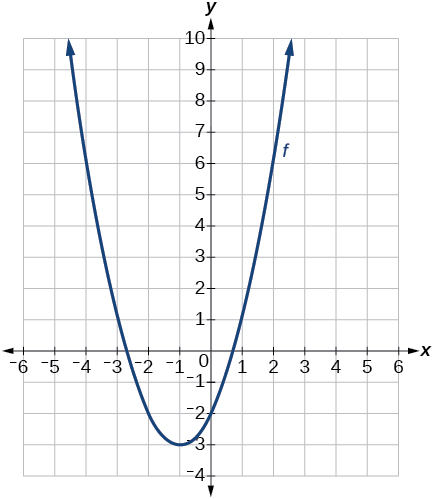$h\left(x\right)=|x-1|+4$

$k\left(x\right)={\left(x-2\right)}^{3}-1$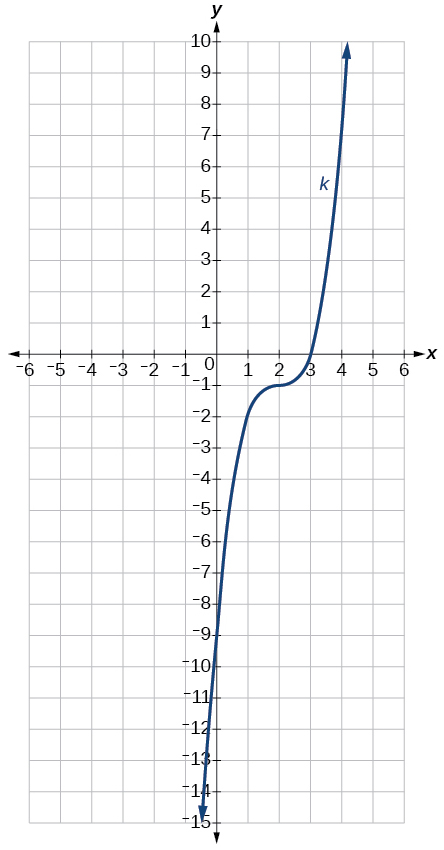$m\left(t\right)=3+\sqrt{t+2}$

## Numeric

Tabular representations for the functions $\text{\hspace{0.17em}}f,\text{\hspace{0.17em}}g,\text{\hspace{0.17em}}$ and $\text{\hspace{0.17em}}h\text{\hspace{0.17em}}$ are given below. Write $\text{\hspace{0.17em}}g\left(x\right)\text{\hspace{0.17em}}$ and $\text{\hspace{0.17em}}h\left(x\right)\text{\hspace{0.17em}}$ as transformations of $\text{\hspace{0.17em}}f\left(x\right).$

 $x$ −2 −1 0 1 2 $f\left(x\right)$ −2 −1 −3 1 2
 $x$ −1 0 1 2 3 $g\left(x\right)$ −2 −1 −3 1 2
 $x$ −2 −1 0 1 2 $h\left(x\right)$ −1 0 −2 2 3

$g\left(x\right)=f\left(x-1\right),\text{\hspace{0.17em}}h\left(x\right)=f\left(x\right)+1$

Tabular representations for the functions $\text{\hspace{0.17em}}f,\text{\hspace{0.17em}}g,\text{\hspace{0.17em}}$ and $\text{\hspace{0.17em}}h\text{\hspace{0.17em}}$ are given below. Write $\text{\hspace{0.17em}}g\left(x\right)\text{\hspace{0.17em}}$ and $\text{\hspace{0.17em}}h\left(x\right)\text{\hspace{0.17em}}$ as transformations of $\text{\hspace{0.17em}}f\left(x\right).$

 $x$ −2 −1 0 1 2 $f\left(x\right)$ −1 −3 4 2 1
 $x$ −3 −2 −1 0 1 $g\left(x\right)$ −1 −3 4 2 1
 $x$ −2 −1 0 1 2 $h\left(x\right)$ −2 −4 3 1 0

For the following exercises, write an equation for each graphed function by using transformations of the graphs of one of the toolkit functions.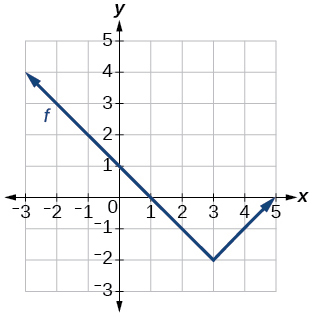$f\left(x\right)=|x-3|-2$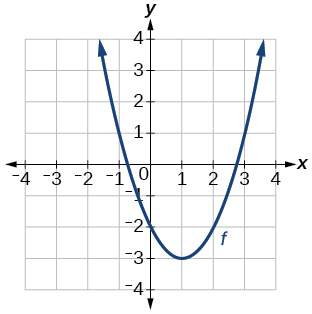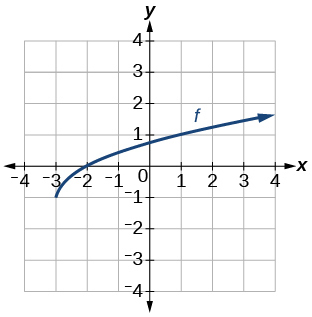$f\left(x\right)=\sqrt{x+3}-1$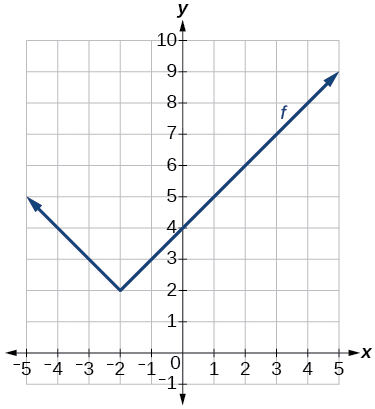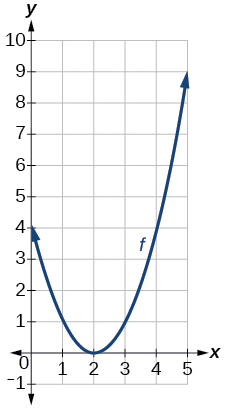$f\left(x\right)={\left(x-2\right)}^{2}$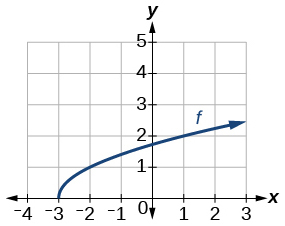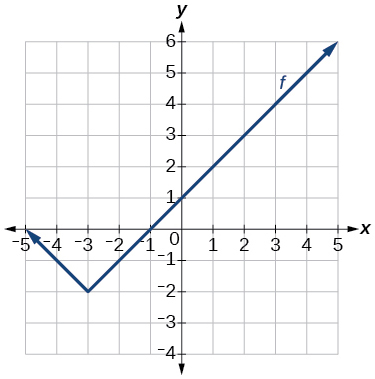$f\left(x\right)=|x+3|-2$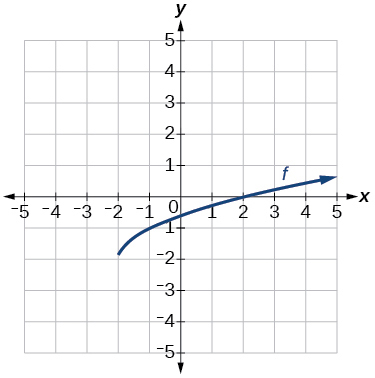For the following exercises, use the graphs of transformations of the square root function to find a formula for each of the functions.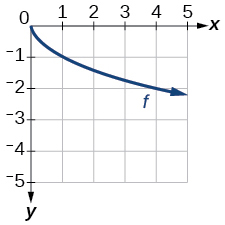$f\left(x\right)=-\sqrt{x}$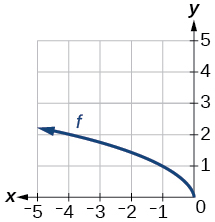For the following exercises, use the graphs of the transformed toolkit functions to write a formula for each of the resulting functions.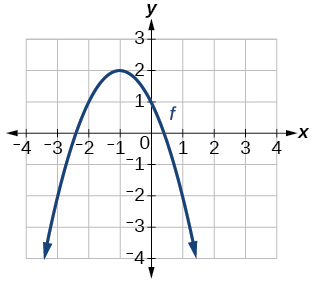$f\left(x\right)=-{\left(x+1\right)}^{2}+2$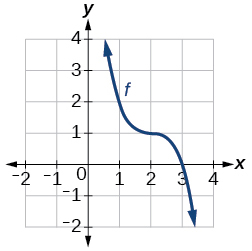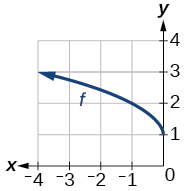$f\left(x\right)=\sqrt{-x}+1$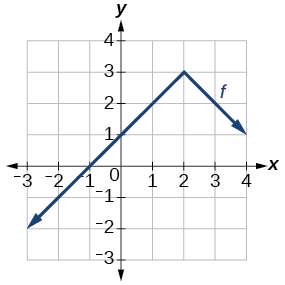For the following exercises, determine whether the function is odd, even, or neither.

$f\left(x\right)=3{x}^{4}$

even

$g\left(x\right)=\sqrt{x}$

$h\left(x\right)=\frac{1}{x}+3x$

odd

$f\left(x\right)={\left(x-2\right)}^{2}$

$g\left(x\right)=2{x}^{4}$

even

$h\left(x\right)=2x-{x}^{3}$

For the following exercises, describe how the graph of each function is a transformation of the graph of the original function $\text{\hspace{0.17em}}f.$

$g\left(x\right)=-f\left(x\right)$

The graph of $\text{\hspace{0.17em}}g\text{\hspace{0.17em}}$ is a vertical reflection (across the $\text{\hspace{0.17em}}x$ -axis) of the graph of $\text{\hspace{0.17em}}f.$

$g\left(x\right)=f\left(-x\right)$

$g\left(x\right)=4f\left(x\right)$

The graph of $\text{\hspace{0.17em}}g\text{\hspace{0.17em}}$ is a vertical stretch by a factor of 4 of the graph of $\text{\hspace{0.17em}}f.$

$g\left(x\right)=6f\left(x\right)$

$g\left(x\right)=f\left(5x\right)$

The graph of $\text{\hspace{0.17em}}g\text{\hspace{0.17em}}$ is a horizontal compression by a factor of $\text{\hspace{0.17em}}\frac{1}{5}\text{\hspace{0.17em}}$ of the graph of $\text{\hspace{0.17em}}f.$

$g\left(x\right)=f\left(2x\right)$

$g\left(x\right)=f\left(\frac{1}{3}x\right)$

The graph of $\text{\hspace{0.17em}}g\text{\hspace{0.17em}}$ is a horizontal stretch by a factor of 3 of the graph of $\text{\hspace{0.17em}}f.$

$g\left(x\right)=f\left(\frac{1}{5}x\right)$

$g\left(x\right)=3f\left(-x\right)$

The graph of $\text{\hspace{0.17em}}g\text{\hspace{0.17em}}$ is a horizontal reflection across the $\text{\hspace{0.17em}}y$ -axis and a vertical stretch by a factor of 3 of the graph of $\text{\hspace{0.17em}}f.$

$g\left(x\right)=-f\left(3x\right)$

For the following exercises, write a formula for the function $\text{\hspace{0.17em}}g\text{\hspace{0.17em}}$ that results when the graph of a given toolkit function is transformed as described.

The graph of $\text{\hspace{0.17em}}f\left(x\right)=|x|\text{\hspace{0.17em}}$ is reflected over the $\text{\hspace{0.17em}}y$ - axis and horizontally compressed by a factor of $\text{\hspace{0.17em}}\frac{1}{4}$ .

$g\left(x\right)=|-4x|$

The graph of $\text{\hspace{0.17em}}f\left(x\right)=\sqrt{x}\text{\hspace{0.17em}}$ is reflected over the $\text{\hspace{0.17em}}x$ -axis and horizontally stretched by a factor of 2.

The graph of $\text{\hspace{0.17em}}f\left(x\right)=\frac{1}{{x}^{2}}\text{\hspace{0.17em}}$ is vertically compressed by a factor of $\text{\hspace{0.17em}}\frac{1}{3},\text{\hspace{0.17em}}$ then shifted to the left 2 units and down 3 units.

$g\left(x\right)=\frac{1}{3{\left(x+2\right)}^{2}}-3$

The graph of $\text{\hspace{0.17em}}f\left(x\right)=\frac{1}{x}\text{\hspace{0.17em}}$ is vertically stretched by a factor of 8, then shifted to the right 4 units and up 2 units.

The graph of $\text{\hspace{0.17em}}f\left(x\right)={x}^{2}\text{\hspace{0.17em}}$ is vertically compressed by a factor of $\text{\hspace{0.17em}}\frac{1}{2},\text{\hspace{0.17em}}$ then shifted to the right 5 units and up 1 unit.

$g\left(x\right)=\frac{1}{2}{\left(x-5\right)}^{2}+1$

The graph of $\text{\hspace{0.17em}}f\left(x\right)={x}^{2}\text{\hspace{0.17em}}$ is horizontally stretched by a factor of 3, then shifted to the left 4 units and down 3 units.

For the following exercises, describe how the formula is a transformation of a toolkit function. Then sketch a graph of the transformation.

$g\left(x\right)=4{\left(x+1\right)}^{2}-5$

The graph of the function $\text{\hspace{0.17em}}f\left(x\right)={x}^{2}\text{\hspace{0.17em}}$ is shifted to the left 1 unit, stretched vertically by a factor of 4, and shifted down 5 units.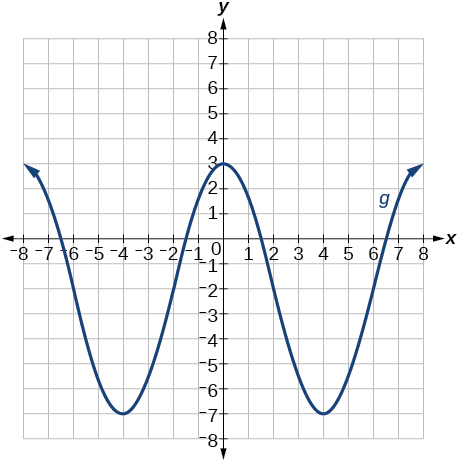$g\left(x\right)=5{\left(x+3\right)}^{2}-2$

$h\left(x\right)=-2|x-4|+3$

The graph of $\text{\hspace{0.17em}}f\left(x\right)=|x|\text{\hspace{0.17em}}$ is stretched vertically by a factor of 2, shifted horizontally 4 units to the right, reflected across the horizontal axis, and then shifted vertically 3 units up.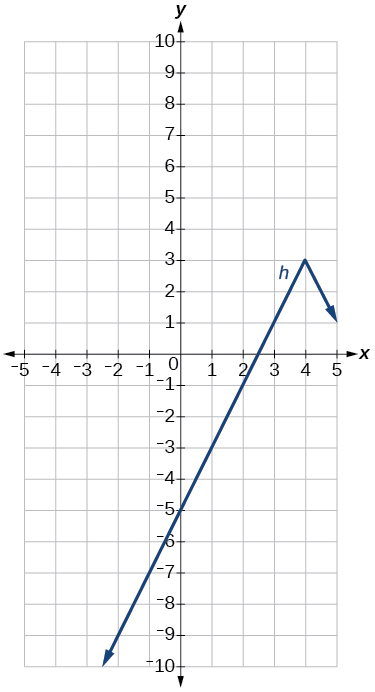$k\left(x\right)=-3\sqrt{x}-1$

$m\left(x\right)=\frac{1}{2}{x}^{3}$

The graph of the function $\text{\hspace{0.17em}}f\left(x\right)={x}^{3}\text{\hspace{0.17em}}$ is compressed vertically by a factor of $\text{\hspace{0.17em}}\frac{1}{2}.$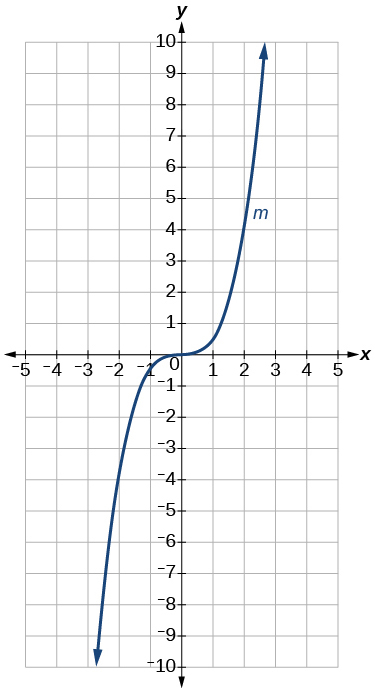$n\left(x\right)=\frac{1}{3}|x-2|$

$p\left(x\right)={\left(\frac{1}{3}x\right)}^{3}-3$

The graph of the function is stretched horizontally by a factor of 3 and then shifted vertically downward by 3 units.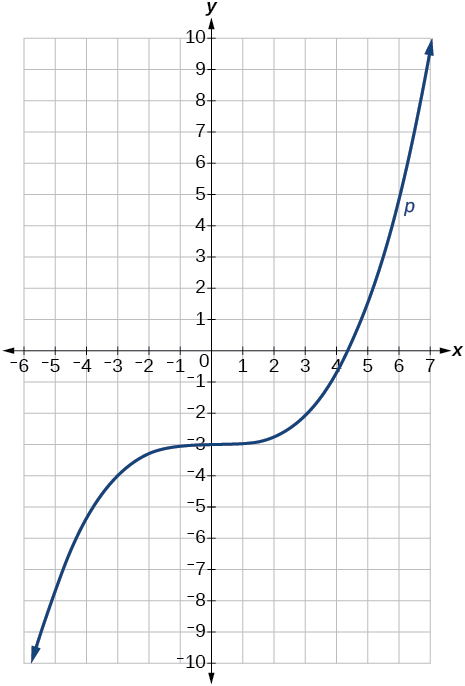$q\left(x\right)={\left(\frac{1}{4}x\right)}^{3}+1$

$a\left(x\right)=\sqrt{-x+4}$

The graph of $\text{\hspace{0.17em}}f\left(x\right)=\sqrt{x}\text{\hspace{0.17em}}$ is shifted right 4 units and then reflected across the vertical line $\text{\hspace{0.17em}}x=4.$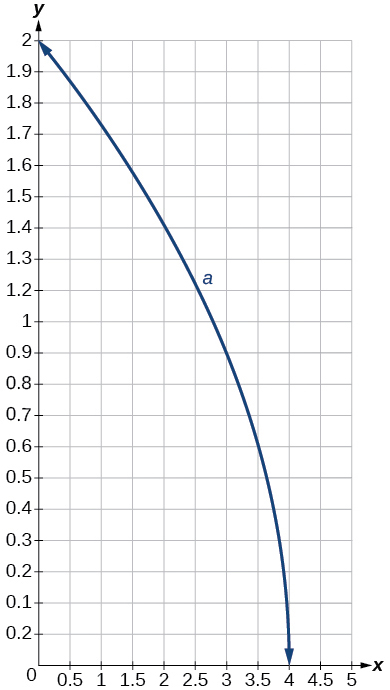For the following exercises, use the graph in [link] to sketch the given transformations.

$g\left(x\right)=f\left(x\right)-2$

$g\left(x\right)=-f\left(x\right)$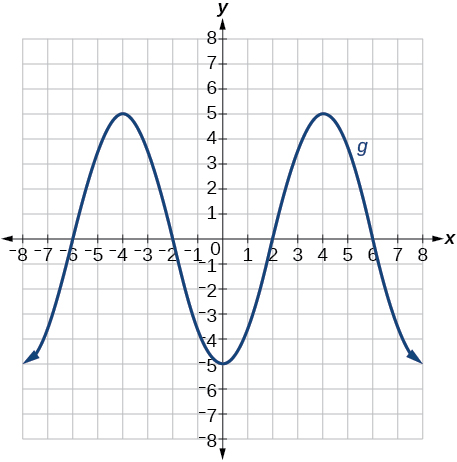$g\left(x\right)=f\left(x+1\right)$

$g\left(x\right)=f\left(x-2\right)$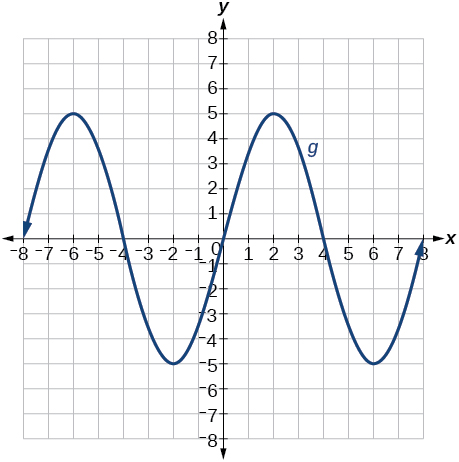1.1 exercise ke all qus
answer and questions in exercise 11.2 sums
how do u calculate inequality of irrational number?
Alaba
give me an example
Chris
and I will walk you through it
Chris
what is a algebra
hiiii
hii
Kundan
hii
Master
what is the identity of 1-cos²5x equal to?
__john __05
Kishu
Hi
Abdel
hi
Ye
hi
Nokwanda
C'est comment
Abdel
Hi
Amanda
hello
SORIE
Hiiii
Chinni
hello
Ranjay
hi
ANSHU
hiiii
Chinni
h r u friends
Chinni
yes
Hassan
so is their any Genius in mathematics here let chat guys and get to know each other's
SORIE
I speak French
Abdel
okay no problem since we gather here and get to know each other
SORIE
hi im stupid at math and just wanna join here
Yaona
lol nahhh none of us here are stupid it's just that we have Fast, Medium, and slow learner bro but we all going to work things out together
SORIE
it's 12
what is the function of sine with respect of cosine , graphically
tangent bruh
Steve
cosx.cos2x.cos4x.cos8x
sinx sin2x is linearly dependent
what is a reciprocal
The reciprocal of a number is 1 divided by a number. eg the reciprocal of 10 is 1/10 which is 0.1
Shemmy
Reciprocal is a pair of numbers that, when multiplied together, equal to 1. Example; the reciprocal of 3 is ⅓, because 3 multiplied by ⅓ is equal to 1
Jeza
each term in a sequence below is five times the previous term what is the eighth term in the sequence
I don't understand how radicals works pls
How look for the general solution of a trig function
stock therom F=(x2+y2) i-2xy J jaha x=a y=o y=b
sinx sin2x is linearly dependent
cr
root under 3-root under 2 by 5 y square
The sum of the first n terms of a certain series is 2^n-1, Show that , this series is Geometric and Find the formula of the n^th
cosA\1+sinA=secA-tanA
Wrong questionByByBy Jonathan LongBy Robert MurphyBy OpenStaxBy OpenStaxBy Cameron CaseyBy OpenStaxBy MldelatteBy Maureen MillerBy Jesenia WoffordBy Richley Crapo